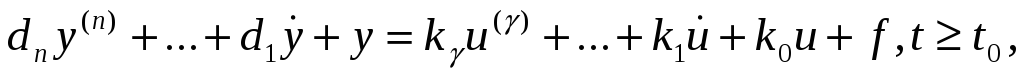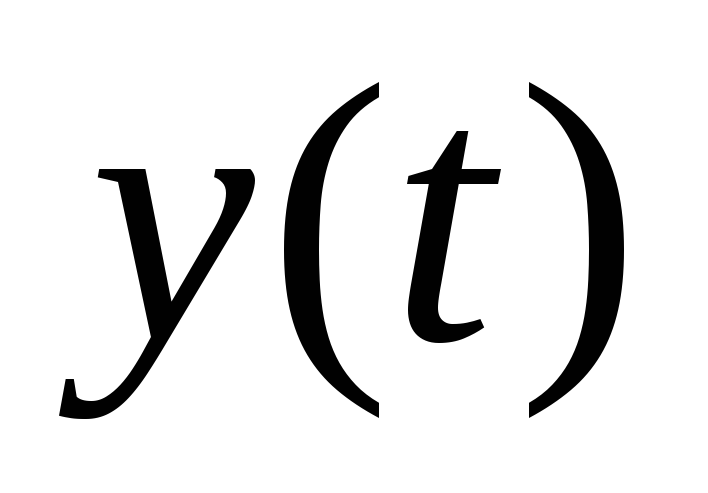# System gamma: the directives of finite-frequency identification Ljubov Mikhailova, Elektrostal Polytechnic Institute of Moscow State Institute of Steel and Alloys

System GAMMA: the directives of finite-frequency identification
Ljubov Mikhailova, Elektrostal Polytechnic Institute of Moscow State Institute of Steel and Alloys (Technological University), Elektrostal, Russia

Albert Alexandrov, Institute of Control Sciences, Moscow, Russia

Abstract

Purpose

To present the results in the area of modern computer-aided control system design tools relating to system identification.

Design/methodology/approach

The finite-frequency identification method was designed for the needs of active identification. The test signal represents the sum of harmonics with automatically tuned (self-tuned) amplitudes and frequencies where the number of harmonics does not exceed the state space dimension of the plant. The self-tuning of amplitudes is carried out to satisfy those requirements on the bounds of the input and output which hold true in the absence of a test signal.

Findings

In this paper the software implementation of finite-frequency identification method in the system GAMMA is considered. GAMMA is the two-level computer-aided design (CAD) tool for identification and controllers algorithms synthesis for linear plants.

Practical implications

The system GAMMA is the complete and really working system.

Originality/value

The new structure of the CAD tool for engineers-developers of control system is proposed and its program implementation is described. The new variant of finite-frequency identification method with self-tuning of identification parameters (test signal and identification time) is considered.

Article type: Research paper

Keywords: Computer-aided design, finite-frequency identification.

1. Introduction

At present, the control theory has at its disposal a number of identification methods for plants specified by linear differential equations. Conventionally, these methods fall into two categories depending on the assumptions on the measurement errors and exogenous disturbances affecting the plant.

The methods of the first class deal with the plants subjected to disturbances of the stochastic nature; i.e., random processes having known statistical characteristics. These are various versions of the method of least squares and the stochastic approximation method; e.g., see well-known monographs (Ljung, 1987).
The second class comprises the identification methods under unknown-but-bounded disturbances (whose statistical properties are not known) such as randomized algorithms (Granichin and Polak, 2003) and finite-frequency identification, see (Alexandrov, 2005).
A somewhat specific position is occupied by the method of instrumental variables (Wong and Polak, 1967). It is developed in the framework of the first class; however, in contrast to the other methods in the class, it is applicable to the problems of the second class so that it is reasonable to consider it as a method of the second category.
The identification process can have passive or active forms. In the passive identification, the measured input of the plant has the meaning of a control action which depends on the control objectives and is not related to identification of the plant. With such an input, identification might not be possible; hence, active identification is often practiced where, in addition to control, the measured input contains an extra component, a so-called test signal aimed at identifying the plant. The finite-frequency identification method was designed for the needs of active identification. The test signal is represented by the sum of harmonics with automatically tuned (self-tuned) amplitudes and frequencies where the number of harmonics does not exceed the state space dimension of the plant. The self-tuning of amplitudes is carried out to satisfy those requirements on the bounds on the input and output which hold true in the absence of a test signal.
The MATLAB-toolbox for finite-frequency identification is described in (Alexandrov, Orlov etc, 2003). In this paper the software implementation of this method in the system GAMMA is considered.
MATLAB (MATLAB, 2001) and GAMMA (Alexandrov, Isakov etc, 2005; Stepanov, 2000) are oriented on different groups of users. MATALB is intended for the scientists primarily. These specialists easily create the programs for solution of real problems of their data domain using a rich spectrum of m-functions. GAMMA is intended for the engineers-developers of control system. The purposes of this group and a small time for Control System development eliminate a capability of their participation in creation of the software for the solution of their problem. Besides the necessity of a profound knowledge of the theory of control also handicaps their work with the MATLAB.
2. Finite-frequency identification

A completely controllable, asymptotically stable plant is described by the following equation:(1)

whereis the measured output,